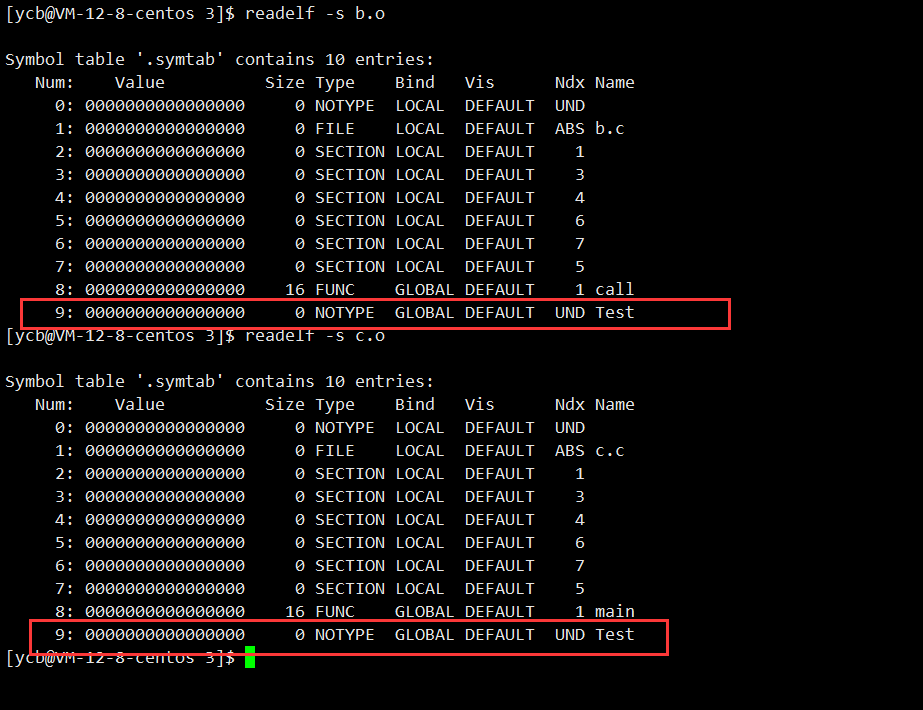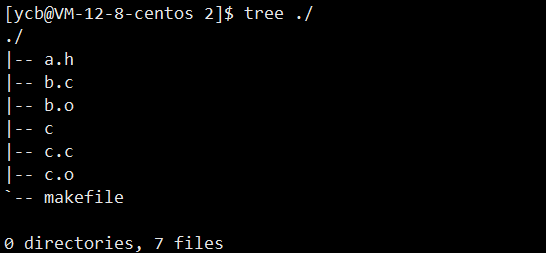﻿ C/C++的关键字之static你了解吗_C 语言_脚本之家
C 语言# C/C++的关键字之static你了解吗

## C语言

### 隐藏

#### 场景演示`a.h`

```#pragma once
#include<stdio.h>
void Test()
{
printf("I am test..\n");
}
```

`b.c`

```#include"a.h"
void call()
{
Test();
}
```

`c.c`

```#include"a.h"
int main()
{
Test();
}
```

`makefile`

```all:c
c:c.o b.o
gcc -o \$@ \$^
c.o:c.c
gcc -c \$^
b.o:b.c
gcc -c \$^
.PHONY:clean
clean:
rm -rf *.o c
```#### 解决方法`a.h`

```#include<stdio.h>
void Test();
```

`a.c`

```#include"a.h"
void Test()
{
cout<<"I am test..."<<endl;
}
```

`makefile`

```all:c
c:c.o b.o a.o
gcc -o \$@ \$^
c.o:c.c
gcc -c \$^
b.o:b.c
gcc -c \$^
a.o:a.c
gcc -c \$^
.PHONY:clean
clean:
rm -rf *.o c
````a.h`

```#pragma once
#include<stdio.h>
static void Test()
{
printf("I am test..\n");
}
```

`代码结构``a.h`

```#pragma once
#include<stdio.h>
static int a =0;
```

`b.h`

```#include"a.h"
void call();
```

`b.c`

```#include"b.h"
void call()
{
a = 1;
printf("a=%d\n",a);
}
```

`c.c`

```#include"b.h"
int main()
{
a=2;
printf("a=%d\n",a);
call();
printf("a=%d\n",a);
}
```### 保持变量内容的持久

• 全局静态变量

• 局部静态变量

```#include<stdio.h>
void test()
{
static int count =0;
count++;
}
int main()
{
for(int i =0 ; i < 10 ; i++ ) test();
}
```

### 默认初始化为0

```#include <stdio.h>
int a;
int main(void)
{
int i;
static char str;
printf("integer: %d;  string: (begin)%s(end)", a, str);
return 0;
}
```

## Cpp

### static类成员变量

• 静态变量属于整个类,所有对象，生命周期在整个程序间运行
• 在类成员函数中，可以随便访问

### static类成员方法

static成员函数，没有this指针，不使用对象就可以调用–>fun::。

```class Date
{
public:
Date(int year=0,int month=1,int day=1)
{
}
void f1()
{
}
static void f2()
{
f1();//没有this指针
}
private:
}
```
```class Date{
public:
void f3()
{
f4();//突破类域+访问限定符就可以访问 Date::f4();/对象.f4()
//类里面是一个整体都在类域中，类里面不受访问限定符限制
}
static void f4()
{
}
private:
};
```

### 单例模式

• 单例模式

1.如何保证全局(一个进程中)只有一个唯一的实例对象

2.如何提供只有一个实例呢？

3.使用场景